# How to Convert Text to Time in Excel

This post will guide you how to convert time string to time in Excel. Assuming that you have a list of text string in your worksheet, and you wish to convert these time string to standard time format, how to do it.

You need to use an formula based on the TIMEVALUE function, the LEFT function, the LEN function and the RIGHT function. Like below:

`=TIMEVALUE(LEFT(B1,LEN(B1)-2)&":"&RIGHT(B1,2))`

Type this formula into a blank cell, and press Enter key on your keyboard to apply it. and drag the AutoFill Handle to other cells to apply this formula.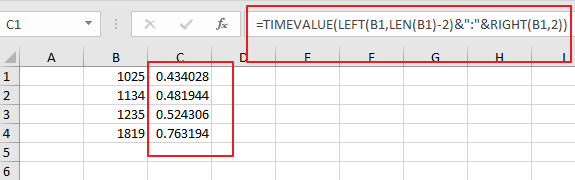Then you need to keep these cells selected, and right click on it, select Format Cells from the context menu list and the Format Cell Dialog will open.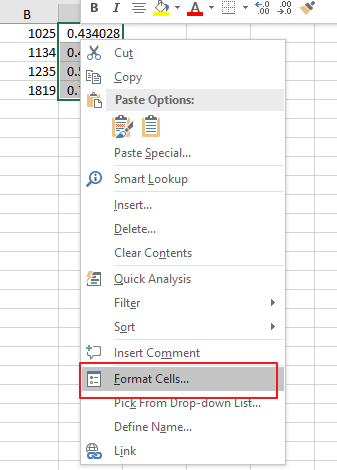Switch to Number tab under Format Cells dialog box, and select Time category from the Category list, and select one time type as you need. click Ok button.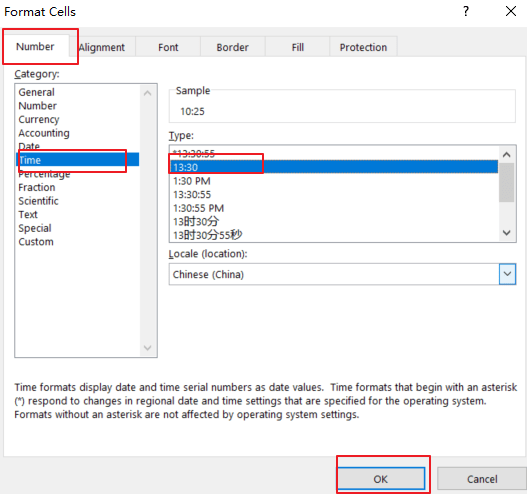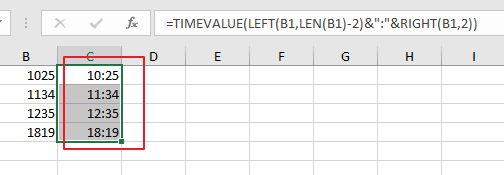If you want to convert date and time string to a data time format in Excel, and you can use the following formula based on the DATE function, the LEFT function, the MID function, the Time Function and the RIGHT function. Like below:

`=DATE(LEFT(B1,4),MID(B1,5,2),MID(B1,7,2))+TIME(MID(B1,10,2),RIGHT(B1,2),0)`

Type this formula into a blank cell and press Enter key on your keyaboard.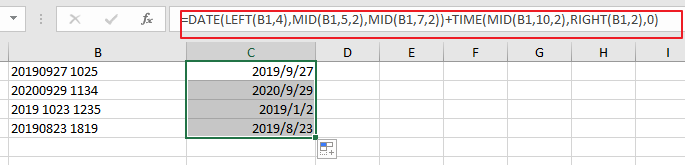Then you need to format those selected cells to a data format as you need.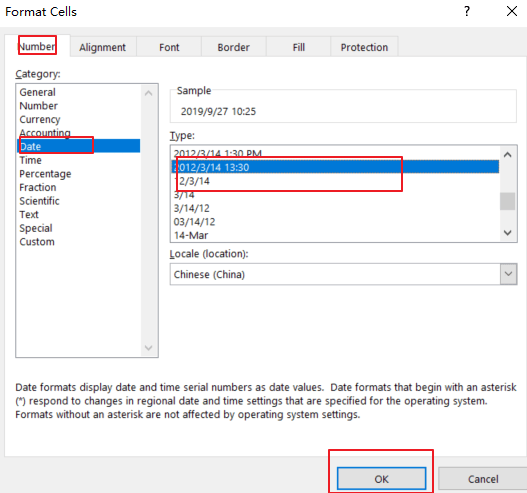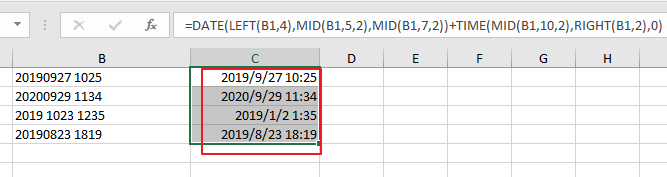### Related Functions

• Excel TIMEVALUE Function
The Excel TIMEVALUE function returns the decimal number of the time represented by a text string. so it will convert a time represented by a text string into an Excel time value.The syntax of the TIMEVALUE function is as below:=TIMEVALUE (time_text)…
• Excel MID function
The Excel MID function returns a substring from a text string at the position that you specify.The syntax of the MID function is as below:= MID (text, start_num, num_chars)…
• Excel LEFT function
The Excel LEFT function returns a substring (a specified number of the characters) from a text string, starting from the leftmost character.The LEFT function is a build-in function in Microsoft Excel and it is categorized as a Text Function.The syntax of the LEFT function is as below:= LEFT(text,[num_chars])…
• Excel RIGHT function
The Excel RIGHT function returns a substring (a specified number of the characters) from a text string, starting from the rightmost character.The syntax of the RIGHT function is as below:= RIGHT (text,[num_chars])…
• Excel TIME function
The Excel TIME function returns a date in serial number format for a particular time (the hour, minute and second).The syntax of the TIME function is as below:= TIME(hour, minute, second)…